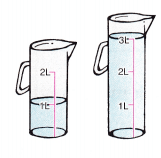# McGraw Hill Math Grade 3 Chapter 9 Lesson 8 Answer Key Problem Solving: Volume and Mass

## McGraw-Hill Math Grade 3 Answer Key Chapter 9 Lesson 8 Problem Solving: Volume and Mass

Solve

Read. Choose the correct operation and solve.

Question 1.
Dr. Torres pours water into 2 containers. How much water did she pour in all?Given,
Dr. Torres pours water into 2 containers.
By seeing the above containers we can add the quantity.
1 + 3 = 4
She poured 4 liters in all.

Question 2.
A nickel has a mass of 5 grams. A dime has a mass of about 3 grams. Shari has 4 nickels and 1 dime. What is the mass of all 5 coins?
Given,
A nickel has a mass of 5 grams.
A dime has a mass of about 3 grams.
Shari has 4 nickels and 1 dime.
5 × 4 = 20
1 × 3 = 3
20 + 3 = 23 grams
The mass of all 5 coins is 23 grams.

Question 3.
Megan used 36 liters of water to fill some fishbowls at the pet shop. She poured 4 liters into each bowl. How many fishbowls did she fill?